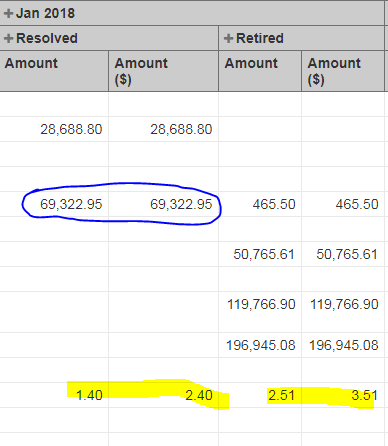# How to add value wherein the custom field contains a comma?

Arithmetic computation are not getting the correct answer when the value contains comma. Example:
1,234.00

I use the following Calculated member formula SUM([Measure].[Amount]) + 1 and those who have comma retains the original value while those that don’t have comma are able to perform the calculated member correctly.

Please refer to the screenshot below:Arithmetic calculations should work for any decimal number. Comma as a thousand separator is used only in a formatting of a decimal number. Please check if totaling works for measure Amount with some default totaling. For example, sums up for All times, or has correct total value when you add Total to Rows.

Your formula uses conditions CASE WHEN and I do not see the whole of it. I can’t see which condition kick in for the particular line.

The formula could work only with Currency dimension expanded to Currency level on Rows or Pages.

I also noticed conditions used in the formula could lead to unpredictable results in some cases:
You are comparing a value in Currency current member (used in the report) with a value in a particular Currency (IDR, MYR, or any other). Addressing the member in the formula works in the similar way as addressing a tuple with default measure:

This expression is a comparison of two tuples:

`````` [Currency].[IDR] =  [Currency].[IDR].CurrentMember
``````

it is the same like this:

``````([Measures].[Issues created],
[Currency].[IDR])
=
([Measures].[Issues created],
[Currency].CurrentMember)
``````

If you would like to compare a currency name of a current member you can use this expression instead:

`````` [Currency].CurrentMember.Name = "IDR"
``````